# How To Find The Formula Of An Exponential Function (3 Methods)

Exponential functions come up often in algebra and calculus.  It is one thing to make a table or graph given the formula for an exponential function, but working backwards is another thing.

So, how do you find the formula of an exponential function?  We can find the formula of an exponential function by using two points on the curve, substituting them into the formula y = abx, and solving the system of two equations in two unknowns. Given a graph or a table of values, we just need to choose two points and use the same method described above.

Of course, we cannot find the formula of an exponential function from one point, since one point (one equation) does not give us enough information to solve for two parameters (the coefficient a and the base b).

In this article, we’ll take a look at the ways you can find the formula of an exponential function.  We’ll also dive into a few examples to make the concepts clear.

Let’s get started.

## How To Find The Formula Of An Exponential Function

To find the formula of an exponential function, all we need is two different points on the curve.  Once we have those two points, we can substitute each pair into the general formula for an exponential function:

• f(x) = abx  (or y = abx)

After we substitute both points, we get two equations in two unknowns (the parameters a and b).  We can then solve the system of equations.

If you are given a table of values for an exponential function, you can simply choose two points and use the same method outlined above.

Calculating Values for X and Y by G...
Calculating Values for X and Y by Graphing | TI-83 Plus and TI-84 Plus Graphing Calculators

If you are given the graph of an exponential function, you can simply choose two points on the curve and use the same method outlined above.

It all comes down to finding two points on the curve and using them to write two equations, then solving the system.

Let’s take a closer look at each method and some examples.

### How To Find The Formula Of An Exponential Function With Two Points

Remember, there are three basic steps to find the formula of an exponential function with two points:

• 1. Plug in the first point into the formula y = abx to get your first equation.
• 2. Plug in the second point into the formula y = abx to get your second equation.
• 3. Solve the system of two equations that you got from steps 1 & 2.  This will give you a and b.

Here are some examples to show how it works.

#### Example 1: How To Find The Formula Of An Exponential Function With Two Points

Let’s say that you are given the points (0, 1) and (2, 9), which lie on the graph of an exponential function.

We know that the general formula for an exponential function is given by:

• f(x) = abx  (or y = abx)

Using the first point (0, 1), we substitute x = 0 and y = 1 to get:

• y = abx
• 1 = ab0
• 1 = a*1
• 1 = a

In this case, the exponent of 0 on b causes it to cancel out, which gives us a = 1.

Now, using the second point (2, 9), we substitute x = 2 and y = 9 to get:

• y = abx
• 9 = b2
• 9 = 1*b2  [since a = 1, as we found before]
• 3 = b  [take the principal (or positive) square root of 9 to get 3]

So, we have b = 3.  Using both a = 1 and b = 3 in the general formula for an exponential function, we get:

• y = abx
• y = 1*3x
• y = 3x

So, the exponential function in this case is y = 3x or f(x) = 3x.

You can see the graph of this function below, which includes the two points (0, 1) and (2, 9).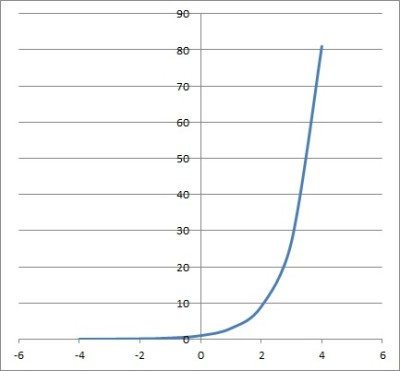The graph of the exponential function y = 3x.

#### Example 2: How To Find The Formula Of An Exponential Function With Two Points

Let’s say that you are given the points (1, 10) and (3, 40), which lie on the graph of an exponential function.

We know that the general formula for an exponential function is given by:

• f(x) = abx  (or y = abx)

Using the first point (1, 10), we substitute x = 1 and y = 10 to get:

• y = abx
• 10 = ab1
• 10 = ab

Now, using the second point (3, 40), we substitute x = 3 and y = 40 to get:

• y = abx
• 40 = ab3

So, our system of two equations in two unknowns is:

• 10 = ab
• 40 = ab3

To solve this system, we will solve for a in the first equation and substitute into the second.

Solving the first equation for a gives us:

• 10 = ab
• 10/b = a

Substituting into the second equation gives us:

• 40 = ab3
• 40 = (10/b)b3
• 40 = 10b3-1
• 40 = 10b2
• 40/10 = b2
• 4 = b2
• 2 = b  [take the principal (or positive) square root of 4 to get 2]

So, we have b = 2.  Substituting into our equation for a, we get:

• 10/b = a
• 10/2 = a
• 5 = a

Using both a = 5 and b = 2 in the general formula for an exponential function, we get:

• y = abx
• y = 5*2x

So, the exponential function in this case is y = 5*2x or f(x) = 5*2x.

You can see the graph of this function below, which includes the two points (1, 10) and (3, 40).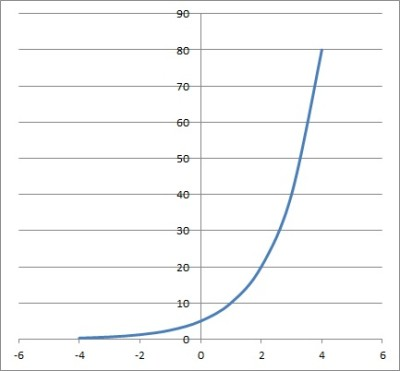The graph of the exponential function y = 5*2x.

### How To Find The Formula Of An Exponential Function Given A Table

If we are given a table for an exponential function, we can just pick any two points.  Then, we can solve for the formula of the exponential function in the same manner as above.

Let’s try an example to see how it works.

#### Example 3: How To Find The Formula Of An Exponential Function Given A Table

Let’s say that you are given the following table of points, which lie on the graph of an exponential function.

Let’s choose the two points (2, 63) and (4, 567).

We know that the general formula for an exponential function is given by:

• f(x) = abx  (or y = abx)

Using the first point (2, 63), we substitute x = 2 and y = 63 to get:

• y = abx
• 63 = ab2

Now, using the second point (4, 567), we substitute x = 4 and y = 567 to get:

• y = abx
• 567 = ab4

So, our system of two equations in two unknowns is:

• 63 = ab2
• 567 = ab4

To solve this system, we will solve for a in the first equation and substitute into the second.

Solving the first equation for a gives us:

• 63 = ab2
• 63/b2 = a

Substituting into the second equation gives us:

• 567 = ab4
• 567 = (63/b2)b4
• 567 = 63b4-2
• 567 = 63b2
• 567/63 = b2
• 9 = b2
• 3 = b  [take the principal (or positive) square root of 9 to get 3]

So, we have b = 3.  Substituting into our equation for a, we get:

• 63/b2 = a
• 63/32 = a
• 63/9 = a
• 7 = a

Using both a = 7 and b = 3 in the general formula for an exponential function, we get:

• y = abx
• y = 7*3x

So, the exponential function in this case is y = 7*3x or f(x) = 7*3x.

You can see the graph of this function below, which includes the two points (2, 63) and (4, 567).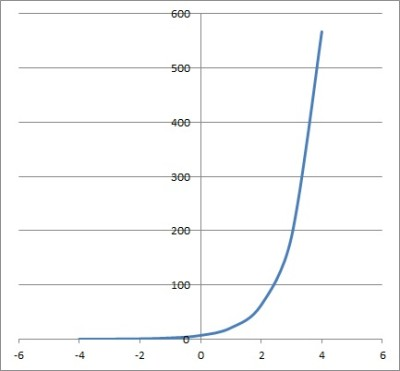The graph of the exponential function y = 7*3x.

### How To Find An Exponential Function From A Graph

If we are given the graph for an exponential function, we can just pick any two points on the curve.  Then, we can solve for the formula of the exponential function in the same manner as above.

Let’s try an example to see how it works.

#### Example 4: How To Find The Formula Of An Exponential Function Given A Table

Let’s say that you are given the following graph of an exponential function.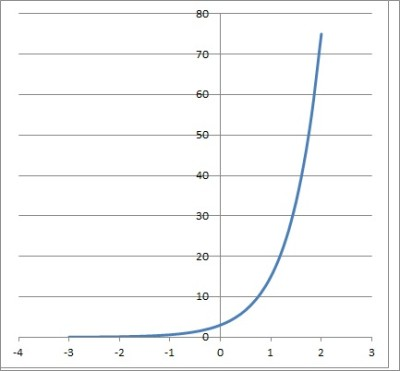The graph of an exponential function. The points (0, 3) and (1, 15) are on the graph.

We can see that the two points (0, 3) and (1, 15) are on the graph.

We know that the general formula for an exponential function is given by:

• f(x) = abx  (or y = abx)

Using the first point (0, 3), we substitute x = 0 and y = 3 to get:

• y = abx
• 3 = ab0
• 3 = a*1
• 3 = a

In this case, the exponent of 0 on b causes it to cancel out, which gives us a = 3.

Now, using the second point (1, 15), we substitute x = 1 and y = 15 to get:

• y = abx
• 15 = 3*b1  [since a = 3, as we found before]
• 15/3 = b  [since a = 1, as we found before]
• 5 = b

So, we have b = 5.  Using both a = 3 and b = 5 in the general formula for an exponential function, we get:

• y = abx
• y = 3*5x

So, the exponential function in this case is y = 3*5x or f(x) = 3*5x.

### How Do You Find The Base Of An Exponential Function?

To find the base “b” of an exponential function, we still need two points, as before.  However, we can use the following formula to find the base b:

• b = (y2/y1)^(1/(x2 – x1))

where the two points on the exponential function curve are (x1, y1) and (x2, y2).

Here is where the above formula comes from:

First, we take the general formula for an exponential function:

• y = abx

Next, we plug in the first point (x1, y1) to get:

• y = abx
• y1 = abx1

Then, we plug in the second point (x2, y2) to get:

• y = abx
• y2 = abx2

Now, we divide y2 by y1 to get:

• y2/y1 = abx2/abx1
• y2/y1 = bx2/bx1  [since the a cancels in the numerator and denominator]
• y2/y1 = bx2-x1 [by the rules of exponents]
• (y2/y1)^(1/(x2 – x1)) = b  [take the root with index x2 – x1 to solve for b]

After we find the base b, we can solve for a by using either of the two points given.

Let’s try an example to see how it works.

#### Example 5: How To Find The Base Of An Exponential Function (From Two Points)

Let’s say we have the points (2, 98) and (3, 686) on an exponential function.  Then our values are:

• x1 = 2
• y1 = 98
• x2 = 3
• y2 = 686

We use these values and the formula from before to find b:

• b = (y2/y1)^(1/(x2 – x1))
• b = (686/98)^(1/(3 – 2))
• b = (7)^(1/(1))
• b = (7)^(1)
• b = 7

Now that we have b = 7, we can use the general formula for an exponential function and the point (2, 98) to find a:

• y = abx
• 98 = a*72  [b = 7, x = 2, y = 98]
• 98 = a*49
• 2 = a

So a = 2.  Using a = 2 and b = 7 in the general formula for an exponential function, we get:

• y = abx
• y = 2*7x

So, the exponential function in this case is y = 2*7x or f(x) = 2*7x.

You can see the graph of this function below, which includes the two points (2, 98) and (3, 686).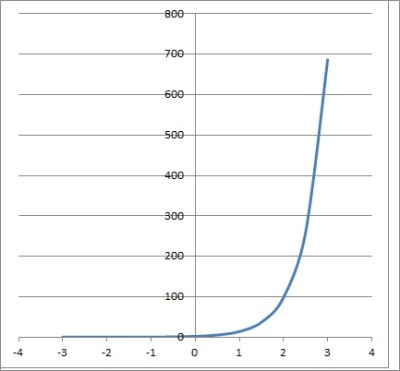The graph of the exponential function y = 2*7x.

## Conclusion

Now you know how to find the formula of an exponential function from two points.  You also know how to choose two points from a table or graph, and how to use a shortcut formula to find the base “b”.

You can learn more about the domain of functions (and how to find it) here.

You can learn more about the natural base e ~ 2.718 here.

I hope you found this article helpful.  If so, please share it with someone who can use the information.

~Jonathon